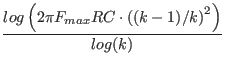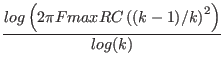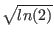Next: Piecewise Linear Up: Tran Functions Previous: Pattern Generation   Contents   Index

### Gaussian Pulse

General Form:
gpulse([v1 v2 td pw per td1 td2 ...] [pattern_spec])
Examples:
vsfq 0 0 gpulse(0 0 20p 2p 0 40p 60p)
vpulse 1 0 gpulse(0 1 100p 5p 100p)

This generates a gaussian pulse signal, and as a special case, as a voltage source will generate single flux quantum (SFQ) pulses. This function applies only to transient analysis, where time is the running variable. The following are the numerical parameters, the pattern_spec is used to specify a patterned pulse train and the syntax is described in 2.15.3.5.

 parameter description default value units v1 base value 0.0 volts or amps v2 pulse peak value v1 volts or amps td delay time 0.0 seconds pw pulse width see description seconds per period 0.0 seconds

Warning: The pulse width is interpreted as the full-width half-maximum in release 4.3.3 and later. In earlier releases, this was taken as the variance'' (width where amplitude is 1/e of the peak). Presently, this interpretation can be coerced by giving a negative pulse width, the absolute value will be used as the variance.

The expression used to generate a pulse is
pw > 0 :

value = v1 + (v2 - v1) . exp(- (4 . ln(2) . (time - td )/pw)2)
pw < 0 :
value = v1 + (v2 - v1) . exp(- ((time - td )/ - pw)2)

The td delay value specifies the time of the initial pulse peak. The pw defines the pulse width, as described above. If the per is given a nonzero value larger than twice the pw, a train of pulses will be generated, the first being at td and at time increments of per thereafter.

Numbers found after the per are taken as additional delays, similar to td. The output is a superposition of pulses found at each delay value (including td). If the per is given a value 0.0, only one pulse per delay value is emitted. If the per specifies a viable period, pulses are emitted at each delay value and increments of per.

If this function is used bare and not part of an expression in a voltage or current source, then the general source instance parameters prm1 etc. map as below. It is possible to read and alter these values using the special vector @device[param] construct, or with the alter and sweep commands. However, there is no sanity checking so bad numbers can cause wild behavior or worse.

 prm1 v1 prm2 v2 prm3 td prm4 pw prm5 per

Periodic pulses can be set to a pattern via the pattern_spec, which can appear following all delay values, if any. The syntax is described in 2.15.3.5.

A single flux quantum (SFQ) pulse, as a voltage applied across an inductor, will induce a single flux quantum of= h/(2e) = 2.06783fWb
where h is Planck's constant, e is the electron charge. With superconductors, the flux that threads superconducting loops is quantized in increments of this value, due to the requirement that the superconducting wave function meet periodic boundary conditions around the loop.

If the pw is not given or given as zero, the source will be configured to produce an SFQ pulse with the given amplitude. Thus, the actual pulse width will be computed internally, with amplitude not zero, as

pw = 2/(abs(v2 - v1))
whereis the flux quantum whose value is given above.

Similarly, if the amplitude is set to zero, i.e., v2 = v1, the amplitude will be computed from the pulse width to yield an SFQ pulse. The computed amplitude is

v2 = v1 + 2/(pw)

If both amplitude and pulse width are set to zero or not given, the full-width half-maximum SFQ pulse width is taken as the TSTEP transient analysis parameter, and the amplitude is computed as above.

In superconducting electronics, single flux quantum pulses are generated and received by logic circuits. A generator of SFQ pulses is therefor a useful item when working with this technology.

Example

* gaussian pulse

v1 1 0 gpulse(0 0 20p 2p 0 40p)
l1 1 2 10p
b1 2 0 100 jj3 area=.2
r2 2 0 2
.tran .1p 100p uic
.plot tran v(1) v(2) i(l1) ysep

* Nb 4500 A/cm2
.model jj3 jj(rtype=1, cct=1, icon=10m, vg=2.8m, delv=0.08m,
+ icrit=1m, r0=30, rn=1.7, cap=1.31p)

In the example, the generator produces two SFQ pulses. The second pulse causes the Josephson junction to emit a flux quantum, the second one from the source is therefor expelled. The inductor current shows the same value before and after the second pulse, as expected.Next: Piecewise Linear Up: Tran Functions Previous: Pattern Generation   Contents   Index
Stephen R. Whiteley 2020-07-23